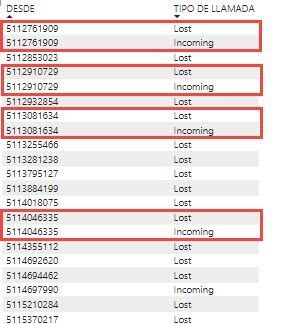cancel
Showing results for
Did you mean:Frequent Visitor

## Calculate measure - count values in same table considering differente categories

I have data from lost and incoming calls and I have to count the lost calls only for number tha don't have incoming calls. If a call from a number is lost, but afterwards is answered (Incoming), that lost call doesn't have to be count. Like this:All the lost calls from numbers never answered have to be count. But those the lost calls in red square doesn't have to be count because that number was answered some time after.

any hint would be veru apreciated

Alberto

1 ACCEPTED SOLUTIONSuper User

=
SUMX (
VALUES ( 'Table'[TESDE] ),
IF ( CALCULATE ( SELECTEDVALUE ( 'Table'[TIPO DE LLAMADA] ) ) = "Lost", 1 )
)

2 REPLIES 2Super User

=
SUMX (
VALUES ( 'Table'[TESDE] ),
IF ( CALCULATE ( SELECTEDVALUE ( 'Table'[TIPO DE LLAMADA] ) ) = "Lost", 1 )
)Frequent Visitor

That's the one! so simple, Thanks